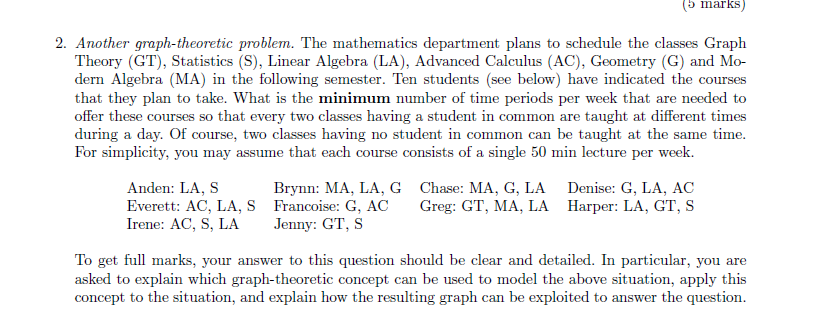# Answered! Another graph-theoretic problem. The mathematics department plans to schedule the classes Graph Theory (GT),…need detail

Don't use plagiarized sources. Get Your Custom Essay on
Answered! Another graph-theoretic problem. The mathematics department plans to schedule the classes Graph Theory (GT),…
GET AN ESSAY WRITTEN FOR YOU FROM AS LOW AS \$13/PAGE

Another graph-theoretic problem. The mathematics department plans to schedule the classes Graph Theory (GT), Statistics (S), Linear Algebra (LA), Advanced Calculus (AC), Geometry (G) and Modern Algebra (MA) in the following semester. Ten students (see below) have indicated the courses that they plan to take. What is the minimum number of time periods per week that are needed to offer these courses so that every two classes having a student in common are taught at different times during a day. Of course, two classes having no student in common can be taught at the same time. For simplicity, you may assume that each course consists of a single 50 min lecture per week. To get full marks, your answer to this question should be clear and detailed. In particular, you are asked to explain which graph-theoretic concept can be used to model the above situation, apply this concept to the situation, and explain how the resulting graph can be exploited to answer the question.

1) There are six classes ( subjects/courses) and 10 students.

2) Graph coloring or node coloring is a model after tabulation of the data.

Anden LA S
Brynn LA MA G
Chase LA MA G
Denise LA G AC
Everett LA S AC
Francoise G AC
Greg LA MA GT
Harper LA S GT
Irene LA S AC
Jenny S GT

3) S and MA , do not have common students. ( one peroid)

AC and GT, do not have common students   (one peroid).

LA and G, have common students. ( 2 peroids are required)

4) Total 4 peroids